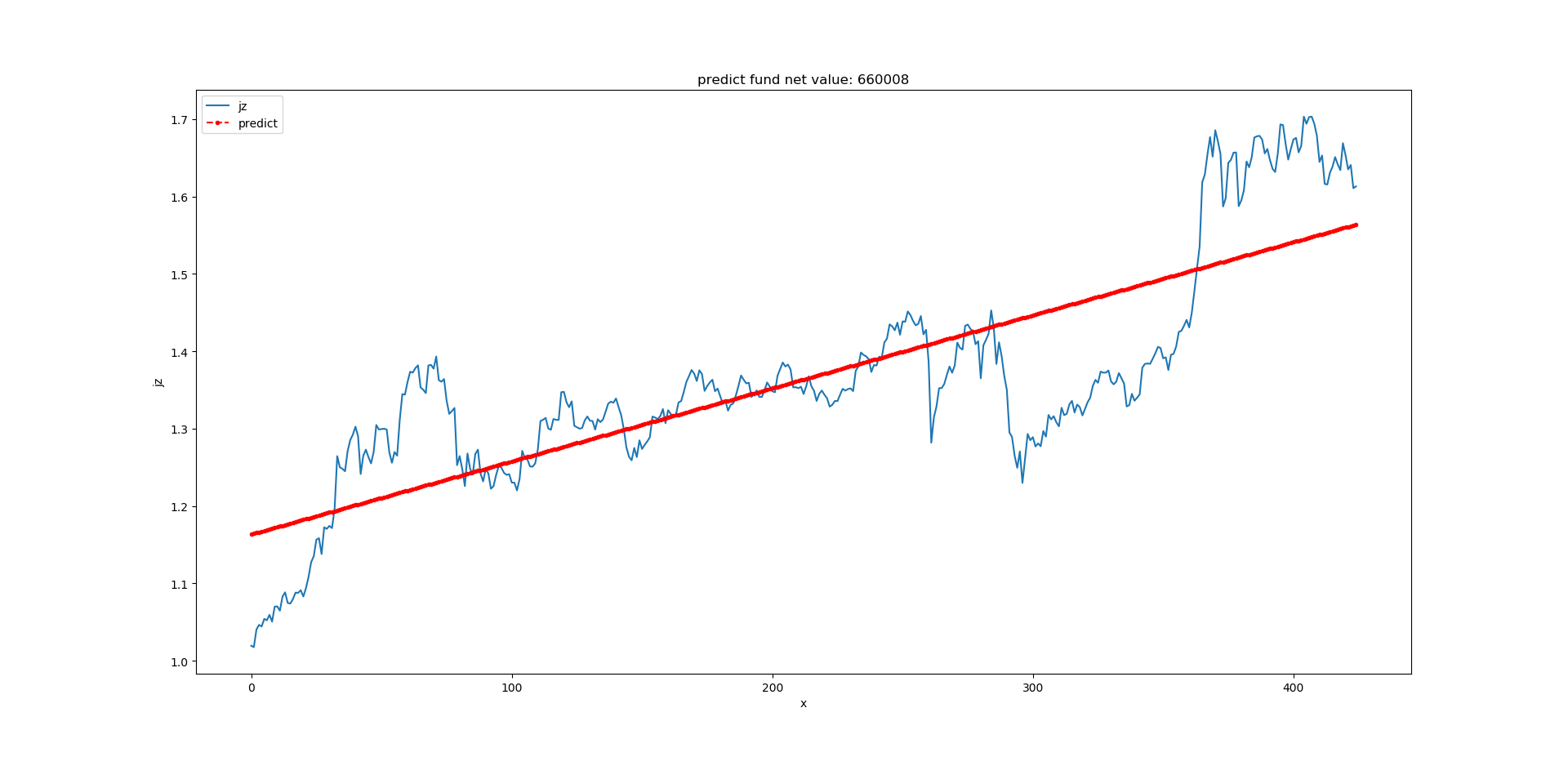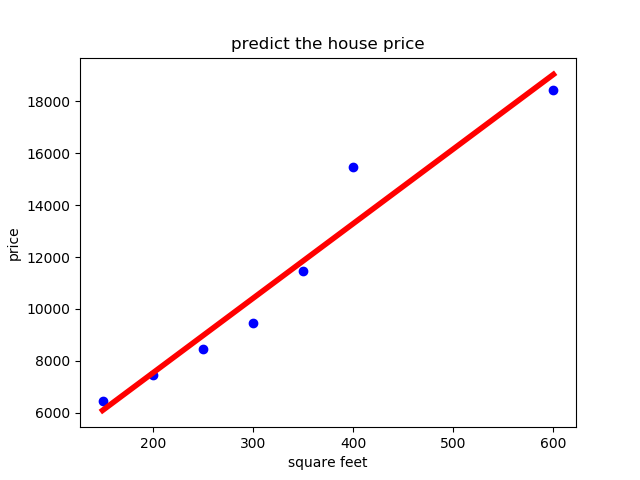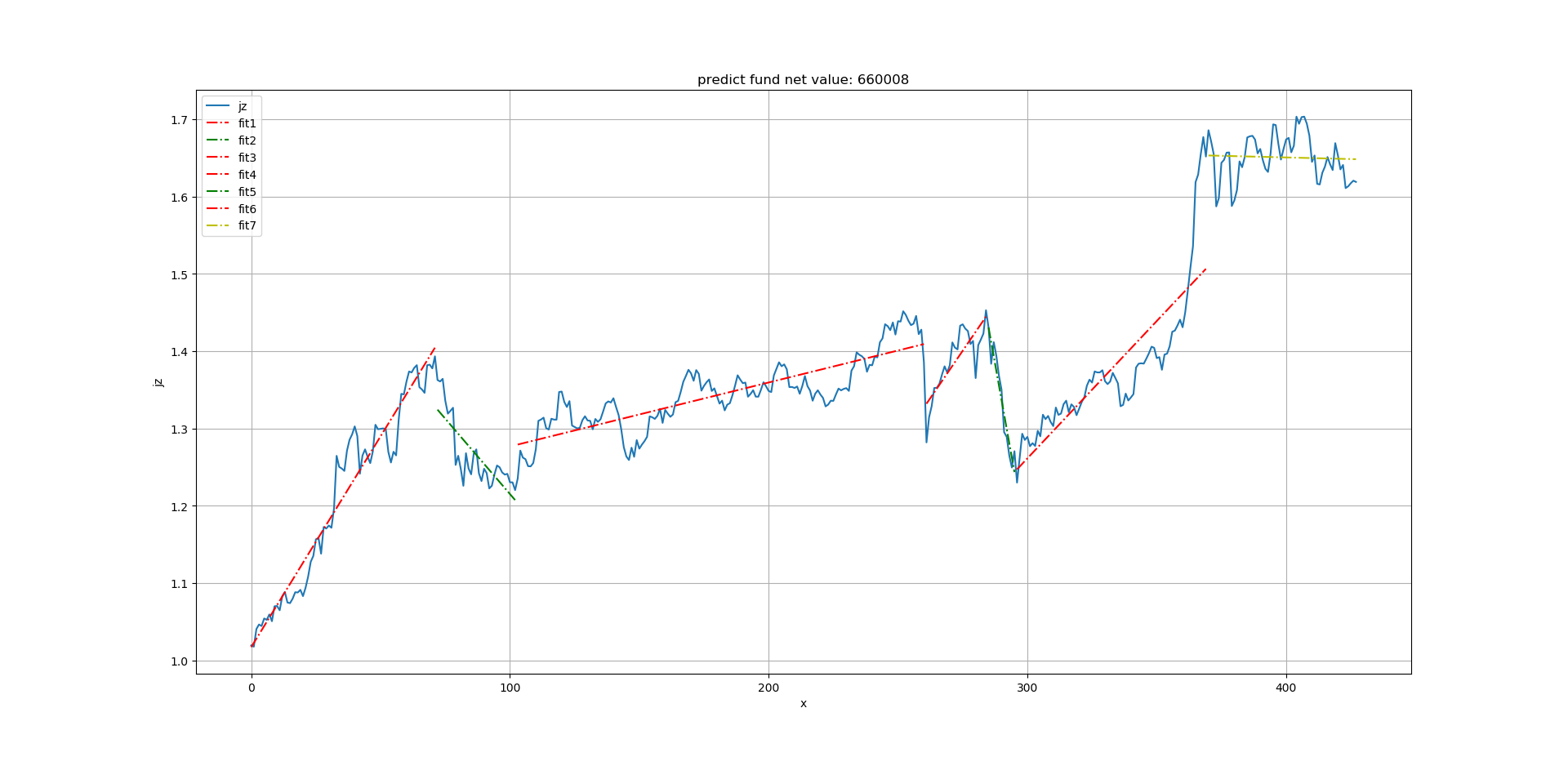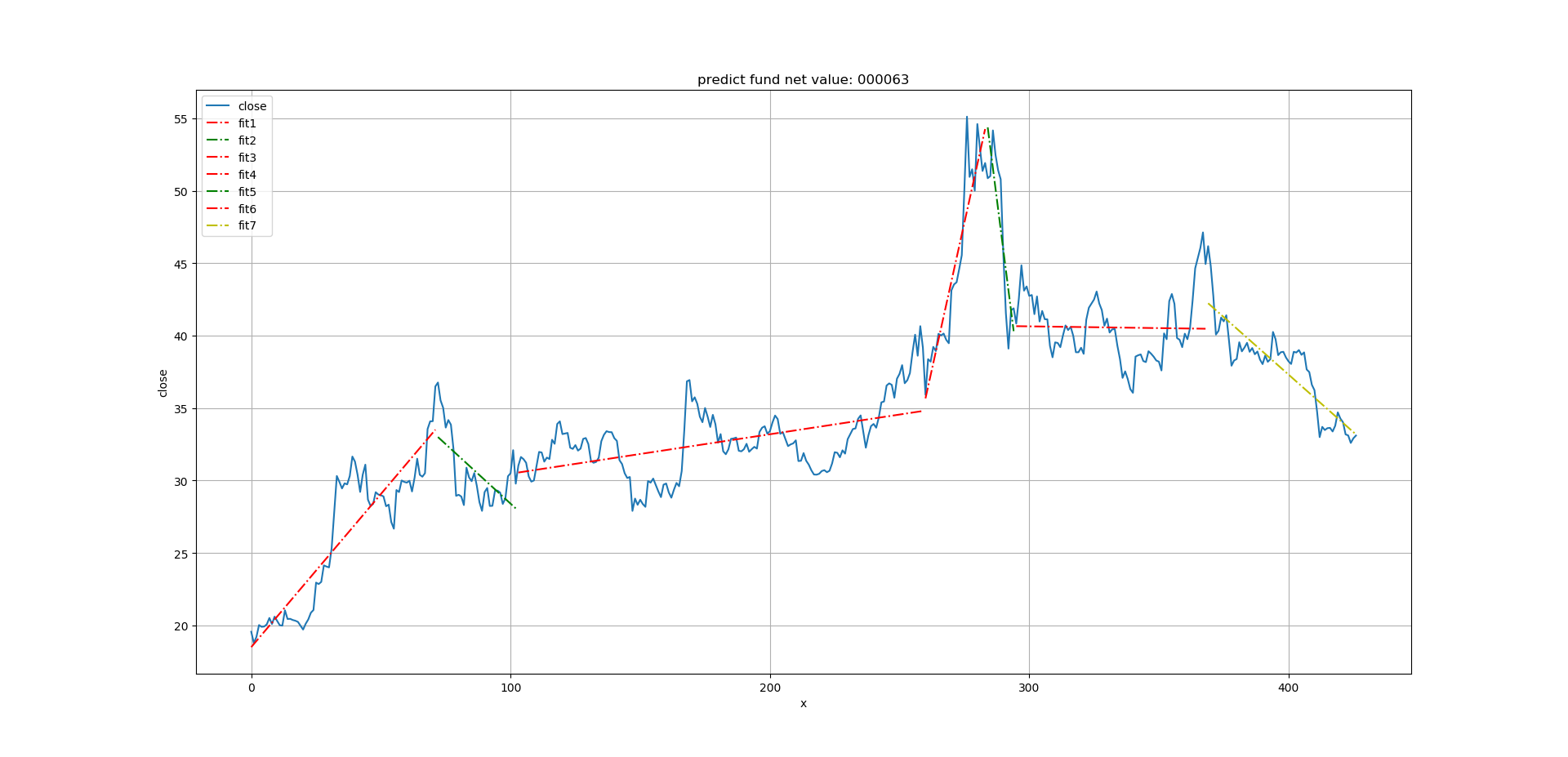• 来自烟水暖的学习笔记回归分析(Regression analysis)回归...回归分析的分类：1） 按自变量的个数，可以分为一元回归，多元回归2）按变量相关性的形状（回归线）是否为直线型，可分为线性回归，非线性回归。下面，...
来自烟水暖的学习笔记回归分析(Regression analysis)回归分析(Regression analysis)，是研究因变量与自变量之间相关性的一种数学方法，并将相关性量化,即得到回归方程。我们可以通过回归方程（回归预测模型）来预测因变量的变化。回归分析的分类：1） 按自变量的个数，可以分为一元回归，多元回归2）按变量相关性的形状（回归线）是否为直线型，可分为线性回归，非线性回归。下面，我们将通过python工具来理解最简单的回归分析，即一元线性回归，并了解回归分析的步骤。想要更多了解回归分析包含的内容，可参考下面内容烟水暖：详细解读Excel回归分析：价格与需求的相关性​zhuanlan.zhihu.com一元线性回归顾名思义，就是只包含一个自变量，且与因变量的关系是呈线性的回归分析，其回归方程（回归模型）可用下面的方程表示：y=bx+a （其中:y为因变量，x为自变量，b为回归系数或斜率，a为截距）简单实例我们将通过分析产品A的单价与销量之间的关系，来预测当产品A的定价为x，市场的需求量y将会是多少，这样我们就能知道该向供应商订购多少产品A。数据如下：确定变量，导入数据2. 探索性数据分析，绘制散点图，观察变量之间关系及趋势变化，确定回归类型#绘制散点图，观察Price,demand的相关性由图可见，Price与Demand具有较强的负相关性，即Price增加，Demand反而减少。我们也可通过下面函数观察Price与Demand的相关系数（相关系数R是描述变量之间的相关性，范围在[-1,1]之间，R>0，则为正相关，R<0，则为负相关；R的绝对值越接近于1，相关性越强，反之，相关性越弱）3. 建立回归模型，并进行模型训练在统计学中，一般我们是通过“最小二乘法”直线拟合来执行线性回归分析。4. 检验模型的拟合程度查看模型的判断系数，判断其拟合度。判断系数为0.8679788013877346，可见模型的拟合程度还是较高的。下面将拟合回归线放入散点图，直观的感受模型的拟合程度:最后查看回归方程的内容:模型包含的内容：参数：fit_intercept： 默认为true,是否计算截距normalize： 是否将数据归一化copy_X：是否复制x的值，默认是复制，否则覆盖原来x的值n_jobs：计算模型使用的作业数，也叫核数属性：coef_:斜率intercept_:截距方法：fit() 拟合线性模型get_params() 获取估计量的参数,即返回上面四个参数的内容predict() 使用模型预测结果score()  返回判定系数 ^2,判定系数描述的是回归方程的拟合优度，值越接近于1,拟合度越高set_params()设置估计量的参数的内容这里，我们只需要拿到下面内容，即可知道回归模型的内容。即Price与Demand呈负相关性，且可用 y=-0.895x+10.128来描述其关系。接下来，我们便可用这个模型来预测需求。5. 使用回归模型预测通过模型的预测方法，我们可预测当产品A的价格为8，或8.2，对应的需求为2.96，2.78.总结通过上面的学习，我们可用了解到最简单的线性回归分析，理解其如何帮我们来分析Price和Demand之间的关系，并由此预测Demand。
展开全文• linear_mod_1.py # coding=utf-8 import os, sys import numpy as np import matplotlib.pyplot as plt ...# python一元线性回归分析实例：预测基金净值 if len(sys.argv) ==2: fcode = sys.argv else: prin
一元线性回归分析实例： 以沪深300指数基金净值为例

基金净值数据格式：

date,jz,ljjz
2019-01-02,1.0194,1.0194
2019-01-03,1.0177,1.0177

linear_mod_1.py

# coding=utf-8
import os, sys
import numpy as np
import matplotlib.pyplot as plt
import pandas as pd
from sklearn.linear_model import LinearRegression

# python一元线性回归分析实例：预测基金净值
if len(sys.argv) ==2:
fcode = sys.argv
else:
print('usage: python linear_mod_1.py fcode ')
sys.exit(1)

if len(fcode) !=6:
print(' fcode is char(6)')
sys.exit(2)

file1 = "./" +fcode +'.csv'
if not os.path.exists(file1):
print(file1 +' is not exists.')
sys.exit(3)

# 用pandas读取csv
df = df[ df['date'] > '2019-01-01']
y = df['jz'].values # 基金净值
x = np.arange(0,len(y),1)

# 构造X列表和Y列表，reshape(-1,1)改变数组形状，为只有一个属性
x = x.reshape(-1,1)
y = y.reshape(-1,1)

# 构造回归对象
model = LinearRegression()
model.fit(x, y)
# 获取预测值
predict_y = model.predict(x)

# 构造返回字典
predictions = {}
predictions['intercept'] = model.intercept_  # 截距值
predictions['coefficient'] = model.coef_   # 回归系数（斜率值）
#predictions['predict_value'] = predict_y
print(predictions)

# 绘图
fig, ax = plt.subplots(figsize=(10,6))
# 绘出已知数据散点图
#plt.scatter(x, y, color ='blue')
# 绘曲线图
ax.plot(x, y, '-', label='jz')  # 基金净值
# 绘出预测直线
ax.plot(x, predict_y, 'r--.', label='predict')
ax.legend(loc='upper left')
plt.title('predict fund net value: ' +fcode)
plt.xlabel('x')
plt.ylabel('jz')
plt.show()



运行 python linear_mod_1.py 660008

{'intercept': array([1.16350283]), 'coefficient': array([[0.00094415]])}参考: https://blog.csdn.net/sinat_25873421/article/details/80791531

展开全文python sklearn
• 本文通过一个简单的例子：预测房价，来探讨怎么用python做一元线性回归分析。 1. 预测房价 房价是一个很火的话题，现在我们拿到一组数据，是房子的大小（平方英尺）和房价（美元）之间的对应关系，如下（csv数据...
本文通过一个简单的例子：预测房价，来探讨怎么用python做一元线性回归分析。

1. 预测房价

房价是一个很火的话题，现在我们拿到一组数据，是房子的大小（平方英尺）和房价（美元）之间的对应关系，如下（csv数据文件）：

No,square_feet,price
1,150,6450
2,200,7450
3,250,8450
4,300,9450
5,350,11450
6,400,15450
7,600,18450

从中可以大致看出，房价和房子大小之间是有相关关系的，且可以大致看出来是线性相关关系。为了简单起见，这里理想化地假设房价只和房子大小有关，那我们在这组数据的基础上，用一元回归分析预测房子的房价。

2. 代码实现

#!usr/bin/env python
#!-*- coding: utf8 -*-
# python一元线性回归分析实例：预测房子价格
import matplotlib.pyplot as plt
import pandas as pd
from sklearn.linear_model import LinearRegression

# 用pandas读取csv
x = data['square_feet']
y = data['price']

# 构造X列表和Y列表，reshape(-1,1)改变数组形状，为只有一个属性
x = x.reshape(-1,1)
y = y.reshape(-1,1)

# 构造回归对象
model = LinearRegression()
model.fit(x, y)
# 获取预测值
predict_y = model.predict(x)

# 构造返回字典
predictions = {}
predictions['intercept'] = model.intercept_  # 截距值
predictions['coefficient'] = model.coef_    # 回归系数（斜率值）
predictions['predict_value'] = predict_y

# 绘出图像
# 绘出已知数据散点图
plt.scatter(x, y, color = 'blue')
# 绘出预测直线
plt.plot(x, predict_y, color = 'red', linewidth = 4)

plt.title('predict the house price')
plt.xlabel('square feet')
plt.ylabel('price')
plt.show()

3. 输出结果参考文章
https://blog.csdn.net/dnxbjyj/article/details/71914943
展开全文• 一元线性回归分析实例：时间序列分段 基金净值数据格式：date,jz,ljjz 2019-01-02,1.0194,1.0194 2019-01-03,1.0177,1.0177 linear_mod_2.py # coding=utf-8 import os, sys import numpy as np import matplotlib...
一元线性回归分析实例：时间序列分段

以沪深300指数基金净值为例
基金净值数据格式：date,jz,ljjz
2019-01-02,1.0194,1.0194
2019-01-03,1.0177,1.0177
linear_mod_2.py

# coding=utf-8
import os, sys
import numpy as np
import matplotlib.pyplot as plt
import pandas as pd
from sklearn.linear_model import LinearRegression

# python一元线性回归分析实例：指数基金净值
if len(sys.argv) ==2:
fcode = sys.argv
else:
print('usage: python linear_mod_2.py fcode ')
sys.exit(1)

if len(fcode) !=6:
print(' fcode is char(6)')
sys.exit(2)

file1 = "./" +fcode +'.csv'
if not os.path.exists(file1):
print(file1 +' is not exists.')
sys.exit(3)

# 用pandas读取csv
df = df[ df['date'] > '2019-01-01']
df.index = pd.to_datetime(df.date)
y = df['jz'].values # 基金净值
x = np.arange(0,len(y),1)

# 构造X列表和Y列表，reshape(-1,1)改变数组形状，为只有一个属性
x = x.reshape(-1,1)
y = y.reshape(-1,1)

# 时间序列分段1
df1 = df[ df['date'] < '2019-04-20']
y1 = df1['jz'].values # 基金净值
x1 = np.arange(0,len(y1),1)
x1 = x1.reshape(-1,1)
y1 = y1.reshape(-1,1)
begin = len(y1)
# 时间序列分段2
dates = pd.date_range('2019-04-20','2019-06-09')
df2 = df[ df.index.isin(dates.values)]
y2 = df2['jz'].values # 基金净值
x2 = np.arange(begin, begin+len(y2),1)
x2 = x2.reshape(-1,1)
y2 = y2.reshape(-1,1)
begin = begin+len(y2)
# 时间序列分段3
dates = pd.date_range('2019-06-10','2020-01-24')
df3 = df[ df.index.isin(dates.values)]
y3 = df3['jz'].values # 基金净值
x3 = np.arange(begin, begin+len(y3),1)
x3 = x3.reshape(-1,1)
y3 = y3.reshape(-1,1)
begin = begin+len(y3)
# 时间序列分段4
dates = pd.date_range('2020-02-03','2020-03-05')
df4 = df[ df.index.isin(dates.values)]
y4 = df4['jz'].values # 基金净值
x4 = np.arange(begin, begin+len(y4),1)
x4 = x4.reshape(-1,1)
y4 = y4.reshape(-1,1)
begin = begin+len(y4)
# 时间序列分段5
dates = pd.date_range('2020-03-06','2020-03-20')
df5 = df[ df.index.isin(dates.values)]
y5 = df5['jz'].values # 基金净值
x5 = np.arange(begin, begin+len(y5),1)
x5 = x5.reshape(-1,1)
y5 = y5.reshape(-1,1)
begin = begin+len(y5)
# 时间序列分段6
dates = pd.date_range('2020-03-21','2020-07-11')
df6 = df[ df.index.isin(dates.values)]
y6 = df6['jz'].values # 基金净值
x6 = np.arange(begin, begin+len(y6),1)
x6 = x6.reshape(-1,1)
y6 = y6.reshape(-1,1)
begin = begin+len(y6)
# 时间序列分段7
df7 = df[ df['date'] > '2020-07-11']
y7 = df7['jz'].values # 基金净值
x7 = np.arange(begin, begin+len(y7),1)
x7 = x7.reshape(-1,1)
y7 = y7.reshape(-1,1)
begin = begin+len(y7)

# 构造回归对象
model = LinearRegression()
model.fit(x1, y1)
Y1 = model.predict(x1) # 获取预测值
model.fit(x2, y2)
Y2 = model.predict(x2)
model.fit(x3, y3)
Y3 = model.predict(x3)
model.fit(x4, y4)
Y4 = model.predict(x4)
model.fit(x5, y5)
Y5 = model.predict(x5)
model.fit(x6, y6)
Y6 = model.predict(x6)
model.fit(x7, y7)
Y7 = model.predict(x7)

# 构造返回字典
predictions = {}
predictions['intercept'] = model.intercept_  # 截距值
predictions['coefficient'] = model.coef_   # 回归系数（斜率值）
#predictions['predict_value'] = Y7
print(predictions)

# 绘图
fig, ax = plt.subplots(figsize=(10,6))
# 绘出已知数据散点图
#plt.scatter(x, y, color ='blue')
# 绘曲线图
ax.plot(x, y, '-', label='jz')  # 基金净值
# 绘出预测直线
ax.plot(x1, Y1, 'r--.', label='fit1')
ax.plot(x2, Y2, 'g--.', label='fit2')
ax.plot(x3, Y3, 'r--.', label='fit3')
ax.plot(x4, Y4, 'r--.', label='fit4')
ax.plot(x5, Y5, 'g--.', label='fit5')
ax.plot(x6, Y6, 'r--.', label='fit6')
ax.plot(x7, Y7, 'y--.', label='fit7')
ax.legend(loc='upper left')
plt.title('predict fund net value: ' +fcode)
plt.xlabel('x')
plt.ylabel('jz')
plt.grid()
plt.show()

以沪深300指数基金净值为例

运行 python linear_mod_2.py 660008python tushare 读取股票数据并存盘

以股票 000063 中兴通讯为例
运行 python stock1.py 000063

将 'jz' 全替换为 'close' 就可以为股票收盘价 做一元线性回归分析

运行 python  linear_mod_2.py 000063展开全文python sklearn
• 本文通过一个简单的例子：预测房价，来探讨怎么用python做一元回归分析python
• 本文通过一个简单的例子：预测房价，来探讨怎么用python做一元线性回归分析。 1. 预测一下房价 房价是一个很火的话题，现在我们拿到一组数据，是房子的大小（平方英尺）和房价（美元）之间的对应关系，见下表（csv数...
• MATLAB一元线性回归分析应用举例 作者：凯鲁嘎吉 - 博客园http://www.cnblogs.com/kailugaji/ huigui.m function [b,bint,r,rint,states,sima2,p,y0,zxqj]=huigui(x,y,x0) %x –p元线性模型自变量的n个观测值...
• x_d)$，其中$x_i$为$\boldsymbol{x}$在第$i$个属性上的取值，线性模型（linear model）试图学得一个通过属性的线性组合来进行预测的函数，即：$\$f(\boldsymbol{x}) = w_1x_1 + w_2x_2 + ... +...
• 一元线性回归可以说是数据分析中非常简单的一个知识点，有一点点统计、分析、建模经验的人都知道这个分析的含义，也会用各种工具来做这个分析。这里面想把这个分析背后的细节讲讲清楚，也就是后面的数学原理。 什么...
• 一元回归分析是啥 上代码 三个库都需要 pip下载： Python机器学习库sklearn的安装 scikit-learn是Python的一个开源机器学习模块，它建立在NumPy，SciPy和matplotlib(绘图)模块之上能够为用户提供各种机器学习...
• 之前的博文讲的是理论。现在需要用到成熟的库，来应用。 Science 科学的工具包 kit # sklearn 命名惯例： ...# LinearRegression 线性回归的类，我们可以使用该类实现线性回归。 # 底层就是使用...机器学习 sklearn
• 2.利用表格1中第一列和第二列做一元回归分析 利用matlab编译程序如下： clear;clc; load('a.mat');%导入数据 n=length(a); X=a(:,1);%提取自变量 X1=[ones(n,1),X];%自变量矩阵前加一列1 Y=a(:,2);%...
• 基于spss的一元线性回归与多元线性回归案例，个人整理出的，包含了部分案例、实验报告、题目，及部分题目答案，适合作为spss、MATLAB等软件数据分析题目联系
• Matlab一元非线性回归分析分析步骤与一元线性回归分析的步骤类似： 大体分为以下几步： （1）绘制x,y的散点图，分析散点图的走势； （2）根据散点图的走势，确定回归方程的具体形式，特别是参数个数的设定和...
• 目标：写清楚一元线性回归的全部过程 步骤： 1. 确定变量variable:independent variable or dependent variable 2. 画散点图确定回归模型scatter diagram 线性相关： 3. 显著性检验：皮尔逊相关系数...
• 剔除异常数据，重新调用regress函数作一元线性回归 1. 读取数据，绘制散点图 ClimateData = xlsread('examp08_01.xls'); % 从Excel文件读取数据 x = ClimateData(:, 1); % 提取ClimateData的第1列，即年平均气温...matlab 读取excel regress
• spss系列——一元线性回归分析与预测实例散点图回归系数二级目录三级目录 本文主要利用某商店记录了12个月的销售收入yyy(亿元)和广告费xxx(亿元)，研究某广告对销售收入的影响，其数据见下表所示。 销售收人与...机器学习
• 回归分析是一种应用广泛的数理统计方法，它是研究变量与变量之间的相关关系，这种关系大致分为两类：确定性关系（能用函数精确描述）和非确定性关系（不能用函数描述）。 变量间的非确定性关系称为相关关系。 在回归...
• 2.1一元线性回归python代码 2.2sklearn代码 3示例 3.1波士顿房价预测 3.2北京房价预测 1.概览 2代码实现 2.1一元线性回归python代码 # -*- coding: utf-8 -*- """ Created on Wed Sep 19 ...回归算法
• 前面，小编和大家一起学习了图形可视化、描述性统计、假设检验等基本...今天，先从SPSS Modeler 进行一元线性回归分析开始学习。 案例：数据文件“房产价格分析V1.xlsx”。 数据展示： 类型节点中定义变量角色： ...
• ... 线性回归分析一个变量与另一个变量的关系的方法。 线性回归的意思就是指x与y之间的关系是线性的。 y = wx+b 求解的步骤： 1. 确定模型。 这里选择y = ...
• # construct model X = tf.placeholder(tf.float32, shape=[None, 1]) W = tf.Variable(tf.random_normal([1, 1]), name='weight') b = tf.Variable(tf.zeros(), name=...python...